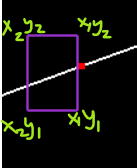# Help Request: Point on Bezier Curve (Collision Detection)

So ive been trying to work on a simple 2d game where the user can move a rectangle through a curve without touching the top and bottom of the square. I am new to opengl and cpp so its kind of hard for me to understand somethings but i am trying to figure out the touch detection for this. I have made the curve using the opengl builtin function evalcoord. I know that i would be needing parametric equations for x and y in terms of t to check if curve is between the y2 and y1 of the square. But i am not sure how to get the t values from the builtin function.You can’t. If you want to find the t value for a given x or y coordinate, you need to find the roots of the corresponding cubic equation (of which there will be 3; at least one root is guaranteed to be real). See Cardano’s formula for a closed-form expression, or e.g. Bisection or Newton’s method for numerical methods (Newton’s converges faster but is more complex).

Another option is essentially the same as bisection but using control points rather than polynomial coefficients: recursively use De Casteljau’s algorithm to split the curve into two halves then check each half. A Bézier curve is bounded by the convex hull of its control points, so you can discard any section where the convex hull doesn’t intersect the line segment.

If you have a Bézier curve segment where all four control points have x coordinates in the interval [x1,x2] and the endpoints have y coordinates on opposite sides of the y coordinate of the horizontal line (y1 or y2), then the line segment is bound to intersect the curve.

If all four control points have y coordinates above the line segment or all four have y coordinates below the line segment, the line segment cannot intersect the curve.

However: one problem with this method is that if one of the line segments is tangent to the curve, the recursion won’t terminate. The endpoints will always lie on one side of the line while the other two control points will line on the other side. So you’ll never get an intersection but nor will you be able to conclude that no intersection occurs. To deal with that, you can either impose an upper bound on the smallest curve segment, or find the maximum or minimum value of the curve by differentiating, solving the quadratic, then substituting back into the cubic.